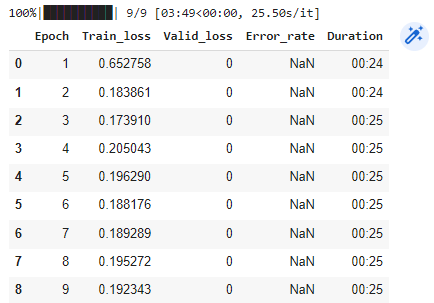# Why are my predictions so bad for the fasterrcnn_resnet50_fpn

Hello, I am using the fastercnn resnet trying to draw some bounding boxes around raccoons. I was able to get the training loop done and also draw the predicted box on some images in the eval state of my saved model. The predictions are pretty bad and I was wondering what I could do to make them better. My training data size is 127 images of raccoons. These are the images with correct boxes taken from the data loader.

``````EPOCHS = 10
BATCH_SIZE = 2

model = torchvision.models.detection.fasterrcnn_resnet50_fpn(pretrained=True) # loading in model as pretrain, IE defualt weights
N_CLASS = 2 #Number of classes we want our model to predict, our case 3 but the background is a class so +1
model.roi_heads.box_predictor = FastRCNNPredictor(INP_FEATURES, N_CLASS)

model.to(device)
params = [p for p in model.parameters() if p.requires_grad]
optimizer = torch.optim.Adam(params) # how our should compute parameters and also how our steps are calculated
lr_scheduler = torch.optim.lr_scheduler.ReduceLROnPlateau(optimizer) # dynamically changes the learning rate

# Training Loop
lossHist = LossAverager()
valLossHist = LossAverager()
column_names = ["Epoch", "Train_loss", "Valid_loss", "Error_rate", "Duration"]
df = pd.DataFrame(columns=column_names)
for epoch in tqdm(range(1, EPOCHS)):

start_time = time()
model.train()  # setting out model to train
lossHist.reset()  # resets our values,averages,sums,counts in lossHist
for images, targets in dl_test:
images = torch.stack(images).to(device)
targets = [{k: v.to(device) for k, v in t.items()} for t in targets]  # sending targets to the GPU
bs = images.shape
loss_dict = model(images, targets)  # passing our model a single batch of images with repective targets
totalLoss = sum(loss for loss in loss_dict.values())
lossValue = totalLoss.item()
lossHist.update(lossValue, bs)
totalLoss.backward()
optimizer.step()
if lr_scheduler is not None:
lr_scheduler.step(totalLoss)

df = df.append({"Epoch": epoch, "Train_loss": lossHist.avg,
"Duration": str(datetime.timedelta(seconds=time() - start_time))[2:7],
"Valid_loss": valLossHist.avg}, ignore_index=True)
torch.save(model.state_dict(), r'model_weights.pth')
df
``````# Loading saved model to inference on

``````model = torchvision.models.detection.fasterrcnn_resnet50_fpn()
N_CLASS = 2
model.roi_heads.box_predictor = FastRCNNPredictor(INP_FEATURES, N_CLASS)
model.eval()
output = model(image)
pprint.pprint(output)
coords = output["boxes"].tolist()